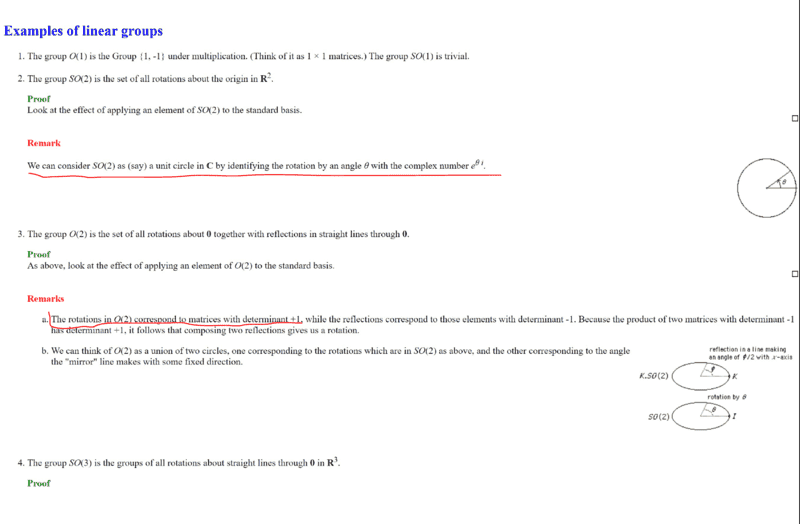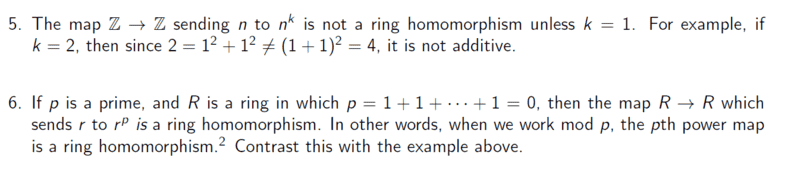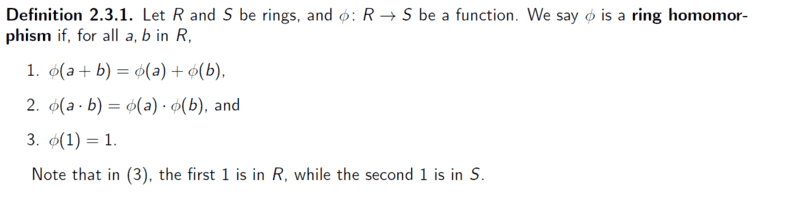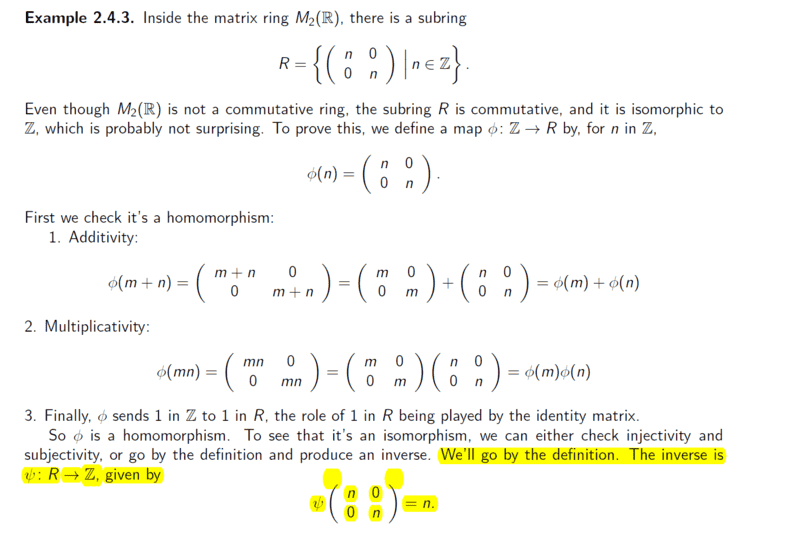# Understanding zero divisors & ##\mathbb{Z_m}## in Ring Theory

• I
• chwala
Actually, no, these are cyclic subgroups of the group of all rotations. This group of all rotations is uncountable and uncountable groups cannot be cyclic.
Let me check on this...

•WWGD
Let me check on this...
And rotation group is a nice example of infinite groups having finite subgroups and having " torsion": Nonzero elements A with ## A^n =0##, which seems counterintuitive. Notice the ring of countable integers being ( very) finitely generated by $$\{1,-1\}$$

⋅⋅Ok, i see your point we cannot pick any item in ##\left [a_0\right]##, in that case we ought to have;

##⋅= 0 mod 9##
##⋅= 0 mod 9##
##⋅= 0 mod 9##

I can also see that,
##⋅= 1 mod 9## implying that #### is an inverse of ####, same to ##7## and ##4##...

You can state that in ##\mathbb{Z_9}## , the Ring of Integers Modulo 9: ##~ ⋅==## . This is written in terms of the residue classes mod 9., which are the actual elements of the ring.
I should have also explicitly enumerated the elements (members) of this ring.
They are: , , , , , , , ,  .
Thus ##\displaystyle \mathbb{Z_9}=\left\{,\,,\,,\,,\,,\,,\,,\,,\,\right\}## .
end of Edit.​

Or as a congruence, you can state that ##~ 6⋅3\equiv 0 \mod 9## .

Or with modular arithmetic: ##~ 6⋅3=0 ## modulo ##9## .

Using the mod operation (a function) you could write: ##~~ (6⋅3) \!\!\!\mod9=0##.

For LaTeX: when writing mod, precede it with a back-slash as in \mod .

##2⋅5=1\mod9## gives ##2⋅5=1\mod9##

##2⋅5=1mod9## gives ##2⋅5=1mod9##

Last edited:
•chwala
And rotation group is a nice example of infinite groups having finite subgroups and having " torsion": Nonzero elements A with ## A^n =0##, which seems counterintuitive. Notice the ring of countable integers being ( very) finitely generated by $$\{1,-1\}$$
I am familiarising with literature to respond amicably to the question, i would like clarification on the highlighted and i also hope that i am looking at the 'correct' literature;

http://campbell.mcs.st-and.ac.uk/~john/MT4521/Lectures/L3.htmlNow on the complex number ##e^{iθ}## this to my understanding is another representation of ##cos θ + i sin θ##. They basically used the conversion from the cartesian coordinates to polar form. We know that ##e^{iθ}=cos θ + i sin θ##. (Euler's equation)
Now on the second part where they indicate that the rotation in a unit circle has determinant of ##1##...this is dependant (in my thinking) on the direction of rotation;
if the direction is say ##90^0## anticlockwise then our matrix of rotation would be;

##\begin{pmatrix}
1& 0\\
0 &1\end {pmatrix}##

if the rotation is say ##270^0## anticlockwise then our rotation matrix would be;
##\begin{pmatrix}
1& 0\\
0 &-1\end {pmatrix}##
giving us determinant values of ##1## and ##-1## respectively. I hope i am getting this right.

Last edited:
To me, typical examples of zero divisors occur in product rings, like ZxZ, where (1,0) x (0,1) = (0,0).

Note also that although in Z/6Z, we have 2.3 = 6 = 0, in fact Z/6Z ≈ Z/2Z x Z/3Z, via the map sending 1 to (1,1), and that 2 and 3 in Z/6Z correspond under this isomorphism to (0,2) and (1,0).

•chwala and Maarten Havinga
To me, typical examples of zero divisors occur in product rings, like ZxZ, where (1,0) x (0,1) = (0,0).

Note also that although in Z/6Z, we have 2.3 = 6 = 0, in fact Z/6Z ≈ Z/2Z x Z/3Z, via the map sending 1 to (1,1), and that 2 and 3 in Z/6Z correspond under this isomorphism to (0,2) and (1,0).
Nice to read this...i think i will try and go through the whole link then get more insight so as to deal with post ##27##.

The insight for me here comes from geometry. If a space S is composed of two pieces, X and Y, then in the ring of functions on S, two functions f and g, such that f vanishes on X, and g vanishes on Y, will have a product that vanishes on all of S. The connection with the previous remark is that this ring of functions on S, is a subring of the product of the ring of functions on X and the ring of functions on Y. Namely a function on S is identified with a pair of functions (f,g), where f is a function on X, g is a function on Y, and f and g agree on the intersection of X and Y. So in algebraic geometry, an algebraic set which is "irreducible", i.e. consists of only one piece, like the x axis, has a ring of functions with no zero divisors, namely just polynomials in X. But a space composed of more than one piece, like the union of the x and y axes, has a ring of functions which does have zero divisors, namely the polynomials in both X and Y, but where the product XY, and any monomial containing XY, is set equal to zero.

I guess it is even simpler when viewed algebraically, as quotient rings. I.e. in a quotient ring, something is set equal to zero, and it just depends on whether or not the thing you set equal to zero is or is not a prime. The ring of functions on the x-axis in the plane, can be viewed as the polynomial ring in X and Y where Y is set equal to zero, and Y is prime. The ring of functions on the union of x and y axes, is the polynomial ring in X and Y, but with the non prime product XY set equal to zero. The ring Z/6Z is the ring Z but with the non prime product 6 = 2.3 set equal to zero, while Z/7Z is Z with the prime 7 set equal to zero. When a product AB is set equal to zero, of course the elements A and B become zero divisors in the quotient ring.

If i understand your question we are looking for ##xy≡0 mod 9## with the condition that ##x≠0 mod 9##and ##y≠0 mod 9## .
Notice that if you use a back-slash ( \ ) with 'mod', the above sentence is rendered as follows:

If I understand your question we are looking for ##xy=0\mod 9## with the condition that ##x≠0\mod 9## and ##y\ne0 \mod 9## .

Now onto my primary reasons for making this reply ...
Now we have,
##[a_0]##=##\{\ldots, -9,0,9,18,27 \ldots\}##
##[a_1]##=##\{\ldots, -8,1,10,19,28 \ldots\}##
if we pick ##[a_0]##=##9## and ##[a_1]##=##1##, then ##[a_0]⋅[a_1]=9⋅1=0 mod 9##

just a minute i check ##1## cannot be a zero divisor in any ring!

Second attempt.

##[a_0]##=##\{\ldots, -9,0,9,18,27 \ldots\}##
##[a_2]##=##\{\ldots, -7,2,11,20,29 \ldots\}##
if we pick ##[a_0]##=##9## and ##[a_2]##=##2##, then ##[a_0]⋅[a_2]=9⋅2=0 mod 9## therefore ##9## and ##2## are zero divisors.
It is improper to state things such as ##[a_0]##=##9## , ##[a_2]##=##2## , etc.
Presumably, by what you have stated early in the quoted post (Post #17), ##a_0## is some integer multiple of ##9## .
Similarly ##a_2## is one of the integers in the set ##\{\dots\, -7,\,2,\,11,\,20,\, \dots \}## . In fact you can say that ##a_2 \in## meaning that
##a_2=9k+2## for some integer, ##k## .

You can pick ##a_0## to be ##9## . That makes ##[a_0]=## . This refers to a set, not an individual integer.

That leads me to mention another misconception you seem to have. There is nothing special about ##a_0,\,a_1,\,a_2,\,a_3,\ ## etc. That is not any sort of general or widely accepted notation. The author of one of the attachments in Post #2, was simply picking a member from one of the residue classes, and using an index (in a subscript) to indicate from which class the pick was made. The point being made was that any particular class can be labeled using any of its members.

One last caution:
Be careful when referring to the ##1## or the ##0## of a ring. Those are, generically speaking, the multiplicative identity and the additive identity, respectively.

For the ring ##\mathbb{Z}_9## , the "1" is ####, This is not the integer ##1##.

Similarly, the zero of this ring is ####. This is not the integer ##0##.

•chwala
Notice that if you use a back-slash ( \ ) with 'mod', the above sentence is rendered as follows:

If I understand your question we are looking for ##xy=0\mod 9## with the condition that ##x≠0\mod 9## and ##y\ne0 \mod 9## .

Now onto my primary reasons for making this reply ...

It is improper to state things such as ##[a_0]##=##9## , ##[a_2]##=##2## , etc.
Presumably, by what you have stated early in the quoted post (Post #17), ##a_0## is some integer multiple of ##9## .
Similarly ##a_2## is one of the integers in the set ##\{\dots\, -7,\,2,\,11,\,20,\, \dots \}## . In fact you can say that ##a_2 \in## meaning that
##a_2=9k+2## for some integer, ##k## .

You can pick ##a_0## to be ##9## . That makes ##[a_0]=## . This refers to a set, not an individual integer.

That leads me to mention another misconception you seem to have. There is nothing special about ##a_0,\,a_1,\,a_2,\,a_3,\ ## etc. That is not any sort of general or widely accepted notation. The author of one of the attachments in Post #2, was simply picking a member from one of the residue classes, and using an index (in a subscript) to indicate from which class the pick was made. The point being made was that any particular class can be labeled using any of its members.

One last caution:
Be careful when referring to the ##1## or the ##0## of a ring. Those are, generically speaking, the multiplicative identity and the additive identity, respectively.

For the ring ##\mathbb{Z}_9## , the "1" is ####, This is not the integer ##1##.

Similarly, the zero of this ring is ####. This is not the integer ##0##.
Thanks @SammyS noted...

Notice that if you use a back-slash ( \ ) with 'mod', the above sentence is rendered as follows:

If I understand your question we are looking for ##xy=0\mod 9## with the condition that ##x≠0\mod 9## and ##y\ne0 \mod 9## .

Now onto my primary reasons for making this reply ...

It is improper to state things such as ##[a_0]##=##9## , ##[a_2]##=##2## , etc.
Presumably, by what you have stated early in the quoted post (Post #17), ##a_0## is some integer multiple of ##9## .
Similarly ##a_2## is one of the integers in the set ##\{\dots\, -7,\,2,\,11,\,20,\, \dots \}## . In fact you can say that ##a_2 \in## meaning that
##a_2=9k+2## for some integer, ##k## .

You can pick ##a_0## to be ##9## . That makes ##[a_0]=## . This refers to a set, not an individual integer.

That leads me to mention another misconception you seem to have. There is nothing special about ##a_0,\,a_1,\,a_2,\,a_3,\ ## etc. That is not any sort of general or widely accepted notation. The author of one of the attachments in Post #2, was simply picking a member from one of the residue classes, and using an index (in a subscript) to indicate from which class the pick was made. The point being made was that any particular class can be labeled using any of its members.

One last caution:
Be careful when referring to the ##1## or the ##0## of a ring. Those are, generically speaking, the multiplicative identity and the additive identity, respectively.

For the ring ##\mathbb{Z}_9## , the "1" is ####, This is not the integer ##1##.

Similarly, the zero of this ring is ####. This is not the integer ##0##.

This part is getting clear to me, in general,
if ##a=b\mod n## then it follows that ##a=b+kn## for some ##k∈ \mathbb{Z}##
For example, we know that
##1≡5\mod 2## then using ##1=5+2k## then the other equivalent values would be given as follows;
##5\mod 2≡7## when ##k=1##.
##5\mod 2≡9## when ##k=2##.
##5\mod 2≡11## when ##k=3##...

This part is getting clear to me, in general,
if ##a=b\mod n## then it follows that ##a=b+kn## for some ##k∈ \mathbb{Z}##
For example, we know that
##1≡5\mod 2## then using ##1=5+2k## then the other equivalent values would be given as follows;
Technically , for the above ##k=-2##, although that's fine.
Also, usually the mod 2 is enclosed in parentheses as in: ##\quad 1≡5\ \ (\mod 2)##

##5\mod 2≡7## when ##k=1##.
##5\mod 2≡9## when ##k=2##.
##5\mod 2≡11## when ##k=3##...
As mathematical function, ##5\mod2## gives the remainder for 5 divided by 2, in the sense of Euclidean Division. In other words, ##5\mod2=1## because ##5=2\cdot2+1## , 2 being the quotient, 1 being the remainder. A rather poor example.

Better: ##7\mod2=1## because ##7=2\cdot3+1## , 3 being the quotient, 1 being the remainder.

Now, if you want to write any of these as congruence mod 2 , then the ##\mod2## usually appears in parentheses at the tail end of the statement.

##5\equiv7\ \ (\mod 2 \ )## means ##7=5+2k\text{, where }k=1## .

A bit cleaner way to consider such a congruence is to see that the following is true.

##5\equiv7\ \ (\mod 2 \ )## means ##7-5=2k\ ## for some integer, ##k##.

•chwala
Technically , for the above ##k=-2##, although that's fine.
Also, usually the mod 2 is enclosed in parentheses as in: ##\quad 1≡5\ \ (\mod 2)##

As mathematical function, ##5\mod2## gives the remainder for 5 divided by 2, in the sense of Euclidean Division. In other words, ##5\mod2=1## because ##5=2\cdot2+1## , 2 being the quotient, 1 being the remainder. A rather poor example.

Better: ##7\mod2=1## because ##7=2\cdot3+1## , 3 being the quotient, 1 being the remainder.

Now, if you want to write any of these as congruence mod 2 , then the ##\mod2## usually appears in parentheses at the tail end of the statement.

##5\equiv7\ \ (\mod 2 \ )## means ##7=5+2k\text{, where }k=1## .

A bit cleaner way to consider such a congruence is to see that the following is true.

##5\equiv7\ \ (\mod 2 \ )## means ##7-5=2k\ ## for some integer, ##k##.
This ought to be pretty obvious to me i.e The Euclidean algorithm...sometimes my thinking is not straight,
anyway,
i note that if;
##7=2\cdot3+1## then we may either have;
##7\mod2=1## or
##7\mod3=1##

In general,
##a=b⋅c+d## then it follows that;
##a\mod c=d##
##a\mod b=d## dependant on either ##b## or ##c## being the quotient and divisor respectively.

This ought to be pretty obvious to me i.e. The Euclidean algorithm...sometimes my thinking is not straight,
anyway,
i note that if;
##7=2\cdot3+1## then we may either have;
##7\mod2=1## or
##7\mod3=1##
True, but in the present discussion, the context was ##\mod 2## .

Thus ##2## is the divisor, ##3## is the quotient, and the remainder is ##1## .

•chwala
I guess it is even simpler when viewed algebraically, as quotient rings. I.e. in a quotient ring, something is set equal to zero, and it just depends on whether or not the thing you set equal to zero is or is not a prime. The ring of functions on the x-axis in the plane, can be viewed as the polynomial ring in X and Y where Y is set equal to zero, and Y is prime. The ring of functions on the union of x and y axes, is the polynomial ring in X and Y, but with the non prime product XY set equal to zero. The ring Z/6Z is the ring Z but with the non prime product 6 = 2.3 set equal to zero, while Z/7Z is Z with the prime 7 set equal to zero. When a product AB is set equal to zero, of course the elements A and B become zero divisors in the quotient ring.
Hey, just confirm that i am getting you right, for Z/7Z we do not have zero divisors! For Z/6Z yes we do have zero divisors.

I can see that under Z/7Z; we have inverses that are commutative under multiplication i.e

##2⋅4=1##
##3⋅5=1##
##1⋅1=1##
##6⋅6=1## only

Am still looking at the literature, i need confirmation on ##6##;Now we know that;My understanding- In reference to 6;
Let ##r=3## and ##p=2##, then it follows that,
##3^2+3^2=18\mod2=0##
##(3+3)^2=36\mod2=0##

satisfies definition 2.3.1 (1)

##3^2⋅ 3^2=81\mod2=1##
##(3⋅3)^2=81\mod2=1##

satisfies definition 2.3.1 (2)

using another example say, ##r=2## and ##p=3##, then it follows that,
##2^3+2^3=16\mod3=1##
##(2+2)^3=64\mod3=1##

satisfies definition 2.3.1 (1)

##2^3⋅ 2^3=64\mod3=1##
##(2⋅2)^3=64\mod3=1##

satisfies definition 2.3.1 (2)

thus the pth power map is a ring homomorphism.

Last edited:
Also here; i need insight on the inverse of a matrix...Are they assuming that in the given symmetric matrix; both ##n## values are units? What if we have non- unit ##n## values? ...then the inverse would not be equal to ##n##.

The p-power is a homomorphism indeed based on your observations. The ##1^p=1## part is trivial but when handing something in do add it.

As to the matrix inverse, there's a slight confusion. They mean the inverse of the homomorphism as a map, not the inverse of the matrix. It's a rather trivial statement.

•chwala
The p-power is a homomorphism indeed based on your observations. The ##1^p=1## part is trivial but when handing something in do add it.

As to the matrix inverse, there's a slight confusion. They mean the inverse of the homomorphism as a map, not the inverse of the matrix. It's a rather trivial statement.
Thanks... we can't have ##1^p=1## because ##p## has to be prime ... unless I am missing something here.

Also here; i need insight on the inverse of a matrix...

View attachment 301452

Are they assuming that in the given symmetric matrix; both ##n## values are units? What if we have non- unit ##n## values? ...then the inverse would not be equal to ##n##.
What do you mean by "both ##n## values" being "units" ?

As @Maarten Havinga states, they are referring to the inverse map .

Show that the map given as ##\psi## is the inverse (as in function inverse) of the map given as ##\phi## .

Last edited:
•chwala
What do you mean by "both ##n## values" being "units" ?

As @Maarten Havinga states, they are referring to the inverse map .

Show that the map given as ##\psi## is the inverse (as in function inverse) of the map given as ##\phi## .
What do you mean by "both ##n## values" being "units" ? ...##n=1##.

Now understood.